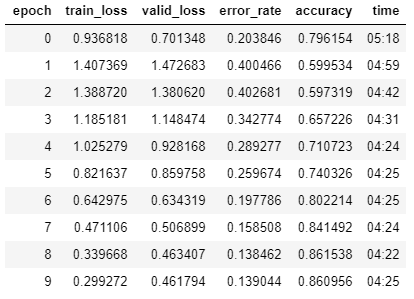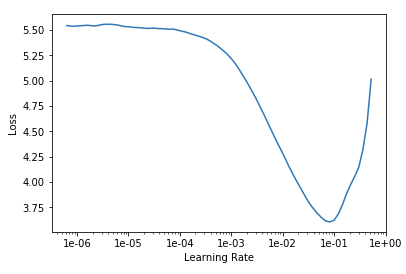# Training & Validation Loss Increases then Decreases

I’m working with the Stanford Dogs 120 dataset, and have noticed that I get the following pattern with ResNet-50 and ResNet-101 where in the second epoch the training and validation loss increases followed by the training and validation loss decreasing in the following epochs.I am using `lr_find()` to select a learning rate where the slope is steepest, and have experimented with different weight `wd` and dropout `ps`, but the pattern still happens. I’m wondering if this is normal or if it means there’s a setting I should change?

Hey, first of all welcome to the community. This shouldn’t be the case according to me. Can you share more of your code?

Sure. I am constructing my data using the datablock api:

``````src = (ImageList.from_folder(img_path, extensions='.jpg')
.split_by_folder(train='train', valid='valid')
.label_from_folder())
.databunch(bs=batchsize)
.normalize(imagenet_stats)
``````

I am using `padding_mode='zeros'` because reflection and border error out and it produces a more accurate results than squishing or cropping. `tfms` are the defaults.Then I create the model, call `lr_find`

``````learn = cnn_learner(data, models.resnet101, metrics=[error_rate, accuracy], path = path)
learn.lr_find()
learn.recorder.plot()
``````And then choose a learning rate between 3e-3 and 6e-3

``````lr = 6e-3
learn.fit_one_cycle(10, lr)
``````

Which results in an output similar to the one I posted. I’ve tried increasing weight decay `wd` and dropout `ps` but it still results in the same pattern.

Did you figure out the reason?

Is your dataset balanced? I have similar issue

Isn’t that the expected behaviour of the one-cycle policy?

01h18m55s in lesson 3 (2019)

4 Likes

TL;DR: your model is working as expected

I saw that you split by folder.
Probably the reason of the behavior you’ve described is the specific validation set you’ve chosen, that is not randomly selected from the same distribution as the training set, but is fixed and pre-defined (maybe holdout).

I’m pretty sure that if you randomly split train and validation set with 80% 20% and re-train the model, you’ll see the accuracy increasing up to the maximum as expected.

That’s right. I forgot about that when I posted the original question. Thanks!